# LCM

Common multiple of three numbers is 3276. One number is in this number 63 times, second 7 times, third 9 times. What are the numbers?

Result

a =  52
b =  468
c =  364

#### Solution:Leave us a comment of example and its solution (i.e. if it is still somewhat unclear...):

Showing 0 comments:Be the first to comment!#### To solve this example are needed these knowledge from mathematics:

Do you want to calculate least common multiple two or more numbers?

## Next similar examples:

1. Buses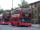At the bus stop is at 10 o'clock met buses No. 2 and No. 9. Bus number 2 runs at an interval of 4 minutes and the bus number 9 at intervals of 9 minutes. How many times the bus meet to 18:00 local time?
2. The smallest numberWhat is the smallest number that can be divided by both 5 and 7
3. LCM of two numberFind the smallest multiple of 63 and 147
4. Lcm simpleFind least common multiple of this two numbers: 140 175.
5. BiketrialKamil was biketrial. Before hill he set the forward gear with 42 teeth and the back with 35 teeth. After how many exercises (rotation) of the front wheel both wheels reach the same position?
6. Apples 2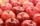How many minimum apples are in the cart, if possible is completely divided into packages of 6, 14 and 21 apples?
7. Dance ensemble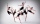The dance ensemble took the stage in pairs. During dancing, the dancers gradually formed groups of four, six and nine. How many dancers have an ensemble?
8. Cents no more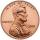Janko bought pencils for 35 cents each. Neither he nor the salesperson had small coins just a whole € 1 coin. At least how many pencils had to buy to pay for the whole euros?
9. Balls groups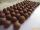Karel pulled the balls out of his pocket and divide it into the groups. He could divide them in four, six or seven, and no ball ever left. How little could be a ball?
10. CherriesCherries in the bowl can be divided equally among 19 or 13 or 28 children. How many is the minimum cherries in the bowl?
11. CagesHonza had three cages (black, silver, gold) and three animals (guinea pig, rat and puppy). There was one animal in each cage. The golden cage stood to the left of the black cage. The silver cage stood on the right of the guinea pig cage. The rat was in the
12. One frame5 picture frames cost € 12 more than three frames. How much cost one frame?
13. Addition of Roman numbersAdded together and write as decimal number: LXVII + MLXIV
14. Primes 2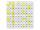Which prime numbers is number 2025 divisible?
15. NumberDetermine unknown number if you know that difference between five times and triple of number is 42.
16. The resultHow many times I decrease the number 1632 to get the result 24?
17. Line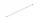How many parts of line divide 5 (different) points that lie on it?